NCERT Exemplar Class 12 Chemistry Chapter 4 Chemical Kinetics are part of NCERT Exemplar Class 12 Chemistry. Here we have given NCERT Exemplar Class 12 Chemistry Chapter 4 Chemical Kinetics.

## NCERT Exemplar Class 12 Chemistry Chapter 4 Chemical Kinetics

Multiple Choice Questions

Question 1. The role of a catalyst is to change
(a) Gibbs energy of reaction (b) enthapy of reaction
(c) activation energy of reaction (d) equilibrium constant
Solution: (c) A catalyst lowers the activation energy of a reaction.

Question 2. In the presence of a catalyst, heat evolved or absorbed during reaction
(a) increases (b) decreases
(c) remains unchanged .(d) may increase or decrease
Solution: (c) There is no effect on heat evolved or absorbed during the reaction in the presence of a catalyst since it does not participate in the reaction.

Question 3. Activation energy of a chemical reaction can be determined by
(a) determining the rate constant at standard temperature
(b) determining the rate constants at two temperatures
(c) determining probability of collision
(d) using catalyst
(b) Activation energy of a chemical reaction is related to rate constant of a reaction at two different temperatures i.e.,K1 and K2  respectively.
Solution:Question 4.Consider the following figure and mark the correct option.(a) Activation energy of forward reaction is E1 + E and product is less stable than reactant.
(b) Activation energy of forward reaction is E1 + E2 and product is more stable than reactant.
(c) Activation energy of both forward and backward reactions is E1 + E2 and reactant is more stable than product.
(d) Activation energy of backward reaction is E, and product is more stable than reactant.
Solution: (a) Ea(forward) = E1 + E2
Since energy of reactants is less than products and the product is less stable than the reactant.

Question 5.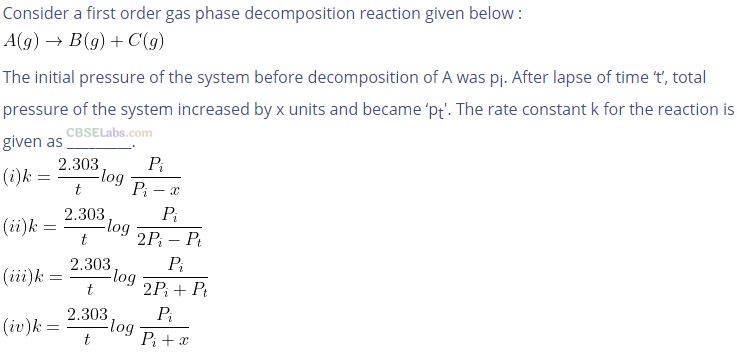Solution: (b) Consider a first order gas phase decomposition reaction: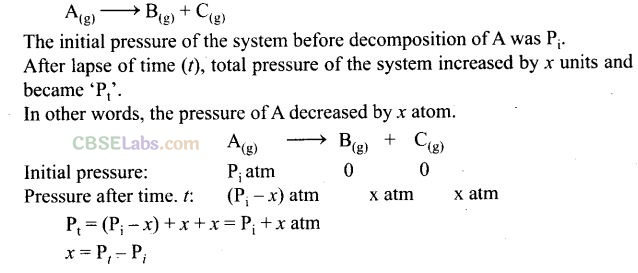Question 6. According to Arrhenius equation rate constant k is equal to Ae-Ea/RT. Which of the following options represents the graph of In k versus ?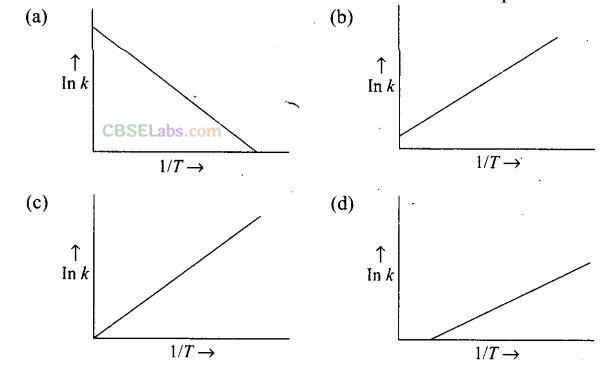Solution:Question 7. Consider the Arrhenius equation given below and mark the correct option. k =Ae-Ea/RT
(a) Rate constant increases exponentially with increasing activation energy _ and decreasing temperature.
(b) Rate constant decreases exponentially with increasing activation energy and decreasing temperature.
(c) Rate constant increases exponentially with decreasing activation energy and decreasing temperature.
(d) Rate constant increases exponentially with decreasing activation energy and increasing temperature.
Solution: (d) K= Ae-Ea/RT from the equation it is clear that value of rate constant k increases exponentially with decrease in activation energy Ea and increase in temperature.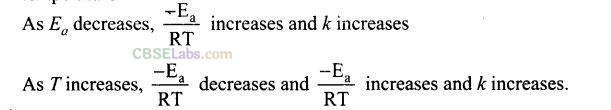Question 8.  A graph of volume of hydrogen released vs time for the reaction between zinc and dil. HCl is shown below. On the basis of this, mark the correct option.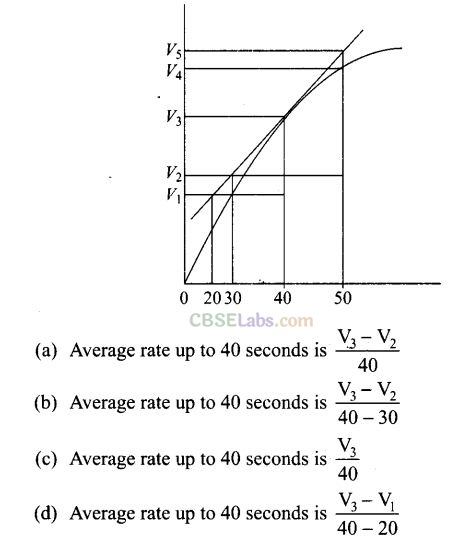Solution: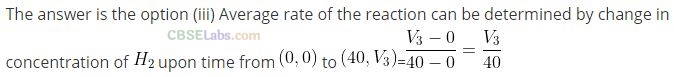Question 9. Which of the following statement is not correct about order of a reaction?
(a) The order of a reaction can be a fractional number.
(b) Order of a reaction is experimentally determined quantity.
(c) The order of a reaction is always equal to the sum of the stoichiometric coefficients of reactants in the balanced chemical equation for a reaction.
(d) The order of a reaction is the sum of the powers of molar concentration of the reactants in the rate law expression.
Solution: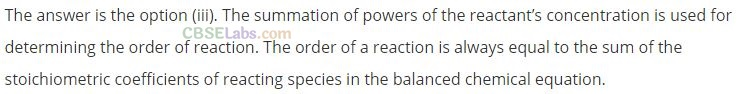Question 10. Consider the graph given in Q. 8. Which of the following option does not show instantaneous rate of reaction at 40th second?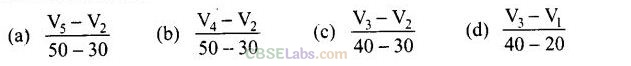Solution:(b) Does not show instantaneous rate of reaction at 40th second.

Question 11. Which of the following statement is correct?
(a) The rate of a reaction decreases with passage of time as the concentration of reactants decreases.
(b) The rate of a reaction is same at any time during the reaction.
(c) The rate of a reaction is independent of temperature change.
(d) The rate of a reaction decreases with increase in concentration of reactant(s).
Solution: (a) The rate of a reaction depends upon the concentration of reactants.

Question 12. Which of the following expressions is correct for the rate of reaction given below?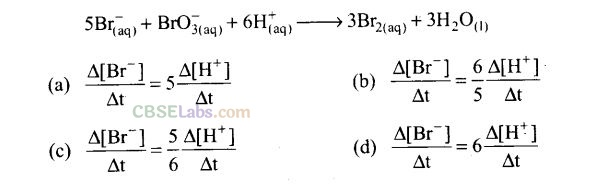Solution:Question 13. Which of the following graph represents exothermic reaction?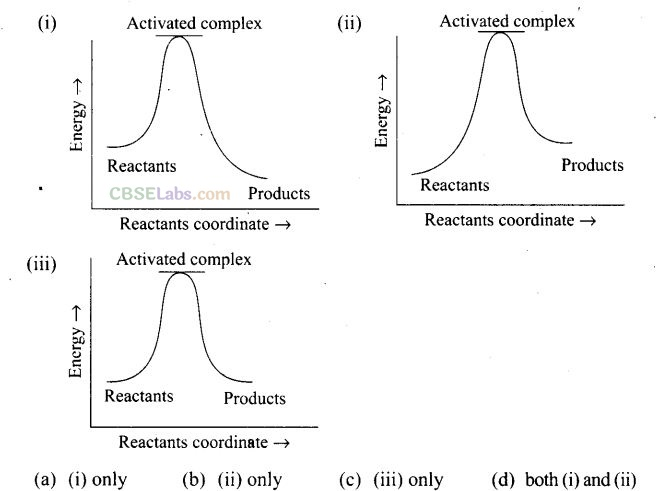Solution:For a exotheramic reaction: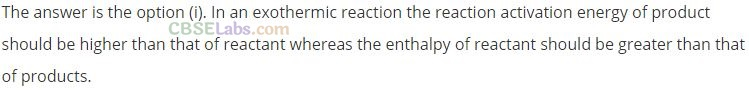Question 14. Rate law for the reaction ‘B’ is doubled, keeping the concentration of ‘A’
constant, the value of rate constant will be
(a) the same (b) doubled (c) quadrupled (d) halved
Solution: (a) Rate constant of a reaction does not depend upon concentrations of the reactants.

Question 15. Which of the following statement is incorrect about the collision theory of chemical reaction?
(a) It considers reacting molecules or atoms to be hard spheres and ignores their structural features.
(b) Number of effective collisions determines the rate of a reaction.
(c) Collision of atoms or molecules possessing sufficient threshold energy results into the product formation.
(d) Molecules should collide with sufficient threshold energy and proper orientation for the collision to be effective.
Solution: (c) According the postulates of collision theory there are the following necessary conditions for any reaction to be occur:
(i) Molecule should collide with sufficient threshold energy.
(ii) Their orientation must be proper.
(iii) The collision must be effective.

Question 16. A first order reaction is 50% completed in 1.26×1014 s. How much time would it take for 100% completion?
(a) 1 26 x 1015 s (b) 2.52×1014 s
(c) 2.52 x 1028 s (d) infinite
Solution: (d) Reaction would be 100% complete only after infinite time which cannot be calculated.

Question 17. Compounds ‘A’ and ‘B’ react according to the following chemical equation.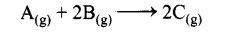Concentration of either ‘A’ or ‘B’ were changed keeping the concentrations of one of the reactants constant and rates were measured as a function of initial concentration. The following results were obtained. Choose the correct option for the rate equations for this reaction.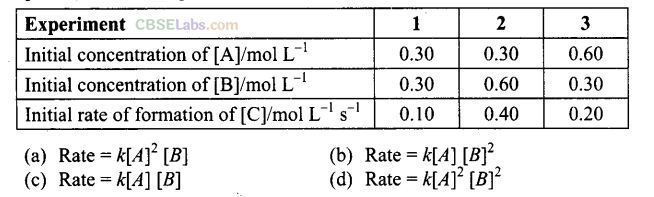Solution: (b)
Rate=K[A]x[B]y
When concentration of B is doubled keeping the concentration of A constant, the rate of formation of C increases by a factor of four. This indicates that the rate of reactions depends upon the square of concentration of B. When concentration of A is doubled, the rate of formation of C also doubles from the initial value. This shows that the rate depends on first power of concentration of A. Hence Rate= K[A]x[B]y.

Question 18. Which of the following statement is not correct for the catalyst?
(a) It catalyses the forward and backward reactions to the same extent.
(b) It alters AG of the reaction.
(c) It is a substance that does not change the equilibrium constant of a reaction.
(d) It provides an alternate mechanism by reducing activation energy between reactants and products.
Solution: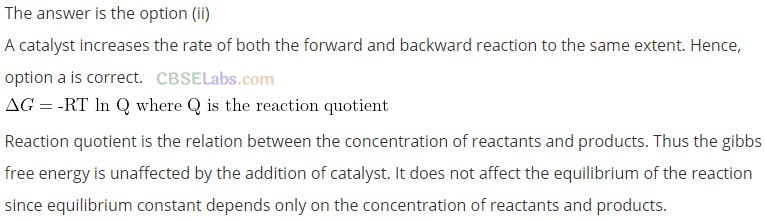Question 19. The value of rate constant of a pseudo first order reaction
(a) depends on the concentration of reactants present in small amount
(b) depends on the concentration of reactants present in excess
(c) is independent of the concentration of reactants
(d) depends only on temperature
Solution: (b) The value of rate constant of a pseudo first order reaction depends on the concentration of reactants present in excess.

Question 20. Consider the reaction A —> B. The concentration of both the reactants and the products varies exponentially with time. Which of the following figures correctly describes the change in concentration of reactants and products with time?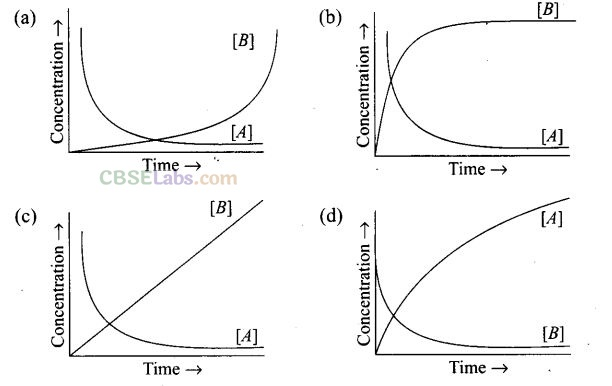Solution: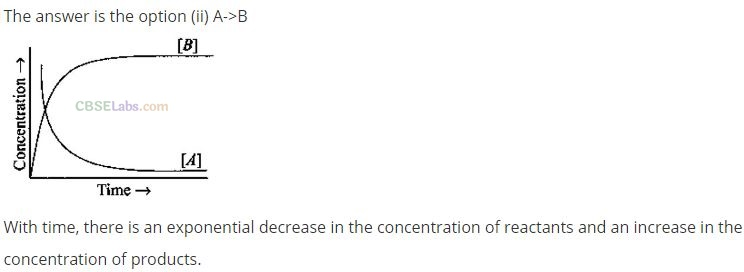More than One Correct Answer Type

Question 21. Rate law cannot be determined from balanced chemical equation if
(a) reverse reaction is involved
(b) it is an elementary reaction
(c) it is a sequence of elementary reactions
(d) any of the reactants is in excess
Solution: (a, c, d) Rate law can be determined from balanced chemical equation if it is an elementary reaction.

Question 22. Which of the following statements are applicable to a balanced chemical equation of an elementary reaction?
(a) Order is same as molecularity
(b) Order is less than the molecularity
(c) Order is greater than the molecularity
(d) Molecularity can never be zero
Solution: (a, d) For an elementary reaction, the order and molecularity are same. Molecularity of a reaction can never be zero.

Question 23. In any uni molecular reaction,
(a) only one reacting species is involved in the rate determining step
(b) the order and the molecularity of slowest step are equal to one
(c) the molecularity of the reaction is one and order is zero
(d) both molecularity and order of the reaction
Solution: (a, b) For any unimolecular reaction, only one reactant is involved in rate determining step and the order and molecularity of rate determining or slowest step are one.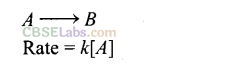Question 24. For a complex reaction
(a) order of overall reaction is same as molecularity of the slowest step
(b) order of overall reaction is less than the molecularity of the slowest step
(c) order of overall reaction is greater than molecularity of the slowest step
(d) molecularity of the slowest step is never zero or non-integer .
Solution: (a, d)
(a) For a complex reaction, order of overall reaction = molecularity of slowest step.
As rate of overall reaction depends upon total number of molecules involved in slowest step of the reaction. Hence, molecularity of the slowest step is equal to order of overall reaction.
(b) Since the completion of any chemical reaction is not possible in the absence of reactants. Hence, slowest step of any chemical reaction must
contain at least one reactant. Thus, molecularity’of the slowest step is never zero or non-integer.

Question 25. At high pressure the following reaction is zero order.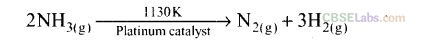Which of the following options are correct for this reaction?
(a) Rate of reaction = Rate constant
(b) Rate of the reaction depends on concentration of ammonia.
(c) Rate of decomposition of ammonia will remain constant until ammonia disappears completely
(d) Further increase in pressure will change the rate of reaction.
Solution: (a, c, d) For a zero order reaction, the rate of reaction is independent of concentration of ammonia.

Question 26. During decomposition of an activated complex
(a) energy is always released (b) energy is always absorbed
(c) energy does not change (d) reactions may be formed
Solution: (a, d) Activated complex is formed at the highest level of energy of the system. It is unstable and decomposes to give products with release of energy. Reactants may be formed if the reaction is reversible.

Question 27. According to Maxwell Boltzmann distribution of energy
(a) the fraction of molecules with most probable kinetic energy decreases at higher temperatures
(b) the fraction of molecules with most probable kinetic energy increases at higher temperatures
(c) most probable kinetic energy increases at higher temperatures
(d) most probable kinetic energy decreases at higher temperatures
Solution: (a, c) Distribution of kinetic energy may be described by plotting a graph of fraction of molecules versus kinetic energy.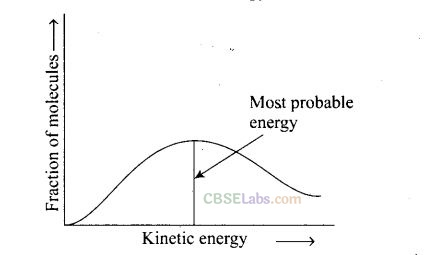Kinetic energy of maximum fraction of molecule is known as most probable kinetic energy. It is important to note that with increase of temperature, peak shifts forward but downward. This means that with increase of temperature
(i) most probable kinetic energy increases.
(ii) the fractions of molecules possessing most probable kinetic energy decreases.

Question 28. In the graph showing Maxwell Boltzmann distribution of energy,(a) area under the curve must not change with increase in temperature
(b) area under the curve increases with increase in temperature
(c) area under the curve decreases with increase in temperature
(d) with increase in temperature curve broadens and shifts to the right hand side
Solution: (a, d) Area under the curve remains same with increase in temperature and with increase in temperature curve broadens and shifts to the right-hand side.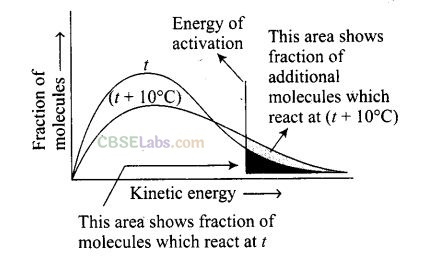Question 29. Which of the following statements are in accordance with the Arrhenius equation?
(a) The rate of a reaction increases with increase in temperature.
(b) The rate of reaction increases with decrease in activation energy.
(c) The rate constant decreases exponentially with increase in temperature.
(d) The rate of reaction decreases with decrease in activation energy.
Solution: (a, b) The rate of reaction increases with temperature. It becomes double with every 10° change. It increases with decrease in activation energy.

Question 30. Mark the incorrect statements.
(a) Catalyst provides an alternative pathway to reaction mechanism.
(b) Catalyst raises the activation energy.
(c) Catalyst lower the activation energy.
(d) Catalyst alters enthapy change of the reaction.
Solution: (b, d) .
(i) As the catalyst is added to the reaction medium rate of reaction increases by decreasing activation energy of molecule. Hence, it follows an alternative pathway.
(ii) Catalyst does not change the enthapy change of reaction. Energy of ‘ reactant and product remain same in both catalysed and  uncatalysed .
reaction.Hence, (a) and (c) are incorrect statements.

Question 31. Which of the following graphs are correct for a zero order reaction?Solution: (a, d) If we plot [R] against t we get a straight line with slope = -k and intercept equal to[R]from the equationQuestion 32. Which of the following graphs are correct for a first order reaction?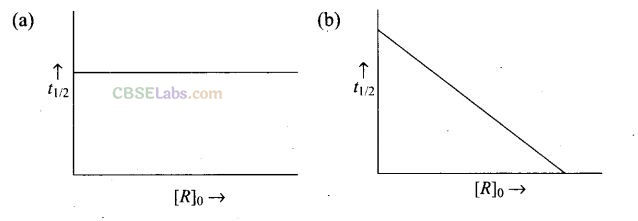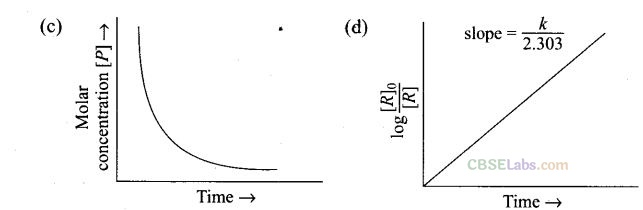Solution: (a, d) For a first order reactionQuestion 33. State a condition under which a biomolecular reaction is kinetically first order reaction.
Solution: Let us consider a biomolecular reaction:
A + B –>Product
Rate = k[A] [B] …………. (i)
When concentration of [B] is taken in large excess, rate law will become Rate = k’ [A]
where, k’ = k[B] ,
The order of reaction will be equal to one.

Question 34. Write the rate equation for the reaction 2A + B–>C if the order of the
reaction is zero.
Solution: 2A+B –>C
Rate = k[A]° [B]°

Question 35. How can you determine the rate law of the following reaction?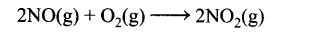Solution: The rate law cqn be determined by measuring the rate of this reaction as a function of initial concentration by keeping the concentration of one of the reactants constant and changing the concentration of other reactant or by changing the concentration of both the reactants. From the concentration dependence of rate, rate law can be determined.

Question 36. For which type of reactions, order and molecularity have the same value?
Solution: Elementary reactions have same value of order and molecularity.

Question 37. In a reaction, if the concentration of reactant A is tripled, the rate of reaction becomes twenty-seven times. What is the order of the reaction?
Solution:Question 38. Derive an expression to calculate time required for completion of zero order reaction. ‘
Solution: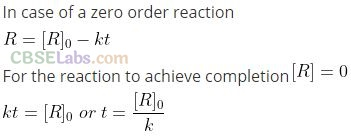Question 39. For a reaction A + B —> Products, the rate law is — Rate = k[A][B]3/2. Can – reaction be an elementary reaction? Explain.
Solution:Question 40. For a certain reaction, large fraction of molecules has energy more than the threshold energy, yet the rate of reaction is very slow. Why?
Solution: Though the reacting molecules may be having energy more than threshold energy, yet they may not be effective due to lack of proper orientation.

Question 41. For a zero order reaction will the molecularity be equal to zero? Explain.
Solution: Molecularity of a reaction can never be equal to zero.

Question 42. For a general reaction A –> B, plot of concentration of A versus time isgiven in figure. Answer the following questions on the basis of this graph.
(i) What is the order of the reaction?
(ii) What is the slope of the curve?
(iii) What are the units of rate constant?
Solution:Question 43. The reaction between H2(g) and O2(g) is highly feasible yet allowing the gases to stand at room temperature in the same vessel does not lead to the formation of water. Explain.
Solution: 2H2(g) +O2(g) –>2H20(l)
This reaction does not take place at room because the activation energy of the reaction is very high.

Question 44. Why does the rate of a reaction increase with rise in temperature?
Solution: At higher temperatures, larger fraction of colliding particles can cross the energy barrier (i.e. the activation energy), which leads to faster rate.

Question 45. Oxygen is available in plenty in air yet fuels do not bum by themselves at room temperature. Explain.
Solution: The activation energy for combustion reactions of fuels is very high at room temperature, therefore, they do not bum by themselves.

Question 46. Why is the probability of reaction with molecularity higher than three very rare?
Solution: The probability of more than three molecules colliding simultaneously is very small. Therefore, the possibility of molecularity being three is very low.

Question 47. Why does the rate of any reaction generally decreases during the course of the reaction?
Solution: The rate of a reaction depends on the concentration of reactants. As the reaction progresses, the concentration of reactants decreases because the reactants start getting converted to products. Hence the rate decreases.

Question 48. Thermodynamic feasibility of the reaction alone cannot decide the rate of the reaction. Explain with the help of one example.
Solution: Thermodynamics feasibility of a reaction depends on Gibbs free energy i.e., AG must be negative for spontaneous process. Kinetic feasibility depends on the activation energy of reaction, the lesser is the activation energy, the greater is the feasibility of reaction, i.e.,This process is thermodynamically feasible but it is very slow due to its high activation energy.

Question 49. Why, in the redox titration of KMnO4versus oxalic acid we heat oxalic acid solution before starting the titration.
Solution: The reaction between KMnO4 and oxalic acid is very slow. By raising the temperature we can increase the rate of reaction.

Question 50. Why cannot molecularity be applicable only for elementary reactions and order applicable for elementary as well as complex reaction?
Solution: Molecularity is the number of molecules involved in the elementary process. It is at least one. Thus, zero molecularity is not possible.

Question 51. Why is molecularity applicable only for elementary reactions and order applicable for elementary as well as complex reaction?
Solution: A complex reaction occurs through several elementary reactions. Numbers of molecules involved in each elementary reaction may be different i.e., the molecularity of each step pi ay be different. Therefore, the molecularity
of overall complex reaction is meaningless. On the other hand, order of a complex reaction is experimentally determined by the slowest step in its mechanism and is therefore, applicable even in the case of complex reactions.

Question 52. Why can we not determine the order of reaction by taking into consideration the balanced chemical equation?
Solution: Balanced chemical equation often leads to incorrect order or rate law. For example, the following reaction appears to be a tenth order reaction.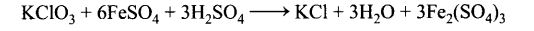However, this is actually a second order reaction. Actually, the reaction is complex and occurs in several steps. The order of such reaction is determined by the slowest step in the reaction mechanism. Order is determined experi¬mentally and gives the actual dependence of observed rate of reaction on the co’ncentration of reactants.

Matching Column Type Questions

Question 53. Match the graph given in Column I with the order of reaction given in Column II. More than one item in Column I may link to the same item of Column II.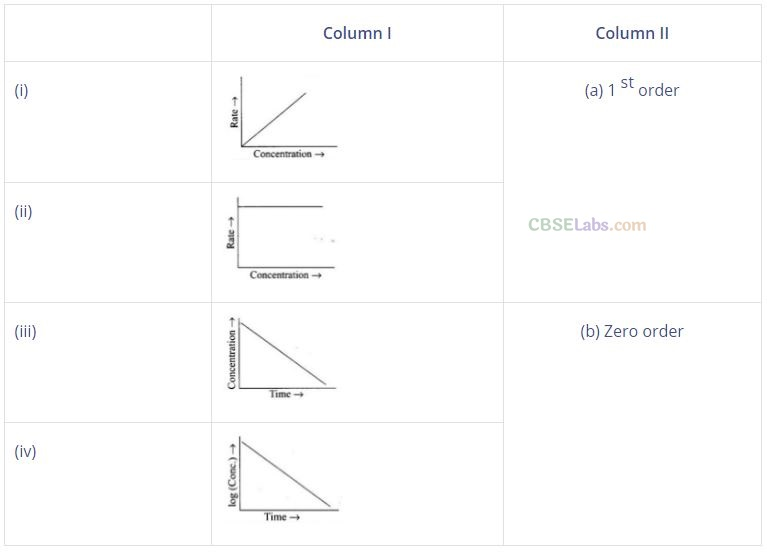Solution:Question 54. Match the statements given in Column I and Column II.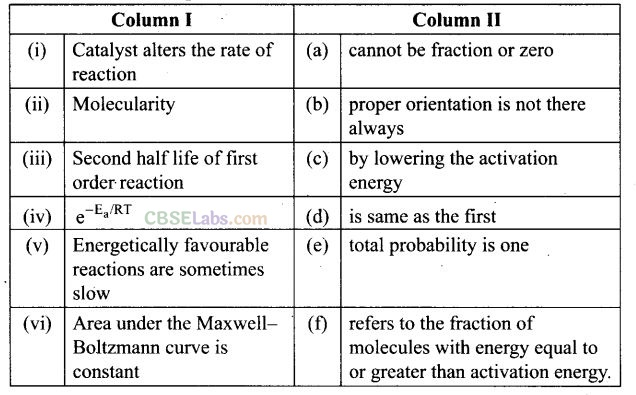Solution: (i —» c), (ii -> a), (iii -> d), (iv -> f), (v -» b), (vi-> e)
(i) Catalyst alters the rate of reaction by lowering activation energy.
(ii) Molecularity cannot be fraction or zero. If molecularity is zero, reaction is not possible.
(iii) Second half-life of first order reaction is same as first because half-life
time is temperature independent. ‘
(iv) e-Ea/RT refers to the fraction of molecules with kinetic energy equal to greater than activation energy.
(v) Energetically favourble reactions are sometimes slow due to improper orientation of molecule cause some ineffective collision of molecules.
(vi) Area under the Maxwell-Boltzmann curve is constant because total probability of molecule taking part in a chemical reaction is equal to one.

Question 55. Match the items of Column I and Column II.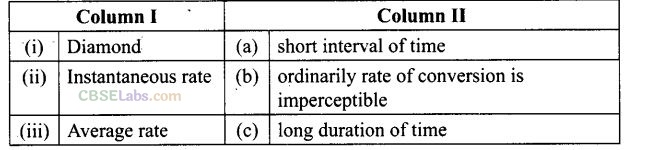Solution: (i ->b), (ii-> a), (iii-> c)
(i) Diamond cannot be converted into graphite under ordinary condition.
(ii) Instantaneous rate of reaction complete at very short span of time.
(iii) Average rate of reaction occurs to a long duration of time.

Question 56. Match the items of Column I and Column II.Solution: (i -> b), (ii -> a), (iii —> d), (iv-> c)
(i) Mathematical expression for rate of reaction is known as rate law.
(ii) Rate of reaction for zero order reaction is equal to rate constant
r = k[A] .
(iii) Unit of rate of reaction is same as that or rate of reaction.
(iv) Order of complex reaction is determined by rate of a reaction, which is slowest.

Assertion and Reason Type Questions

In the following questions, a statement of Assertion (A) followed by a statement of Reason (R) is given. Choose the correct option out of the following choices:
(a) Both Assertion and Reason are correct and the reason is the correct explanation of Assertion.
(b) Both assertion and reason are correct but Reason is not the correct explanation of Assertion
(c) Assertion is correct but Reason is incorrect.
(d) Both Assertion and Reason are incorrect.
(e) Assertion is incorrect but Reason is correct.

Question 57. Assertion (A): Order of the reaction can be zero or fractional.
Reason (R): We cannot determine order from balanced chemical equation.
Solution: (b) Order of a reaction may be zero or fractional. Order can be determined by rate law expression.

Question 58. Assertion (A): Order and molecularity are same.
Reason (R): Order is determined experimentally and molecularity is the sum of the stoichiometric coefficient of rate determining elementary step.
Solution: (e) Order and molecularity may not be necessarily same. Order is determined experimentally but molecularity is calculated using balanced stoichiometric equation.

Question 59. Assertion (A): The enthapy of reaction remains constant in the presence of a catalyst.
Reason (R): A catalyst participating in the reaction, forms different activated complex and lowers down the activation energy but the difference in energy of reactant and product remains the same.
Solution: (a) AH = Activation Energy of forward reaction – Activation Energy of reverse reaction.
Catalyst does not alter heat of reaction because it affects activation energy of forward and reverse reactions equally.

Question 60. Assertion (A): All collision of reactant molecules lead to product formation. Reason (R): Only those collisions in which molecules have correct orientation and sufficient kinetic energy lead to compound formation.
Solution: (e) Every collision among reactant molecules does not lead to the formation of product. Only effective collision brings out the formation of product.

Question 61. Assertion (A): Rate constants determined from Arrhenius equation are fairly accurate for simple as well as complex molecules.
Reason (R): Reactant molecules undergo chemical change irrespective of their orientation during collision.
Solution: (c) According to Arrhenius equation (k =Ae-Ea/RT); it is found almost accurate for single as well as complex reaction.
However, orientation is essential for the reactant molecules participating in the reaction.

Question 62. All energetically effective collisions do not result in a chemical change. Explain with the help of an example.
Solution: Only effective collision leads to the formation of products. It means that collisions in which molecules collide with sufficient kinetic energy (called threshold energy = activation energy + energy possessed by reacting species). And proper orientation lead to a chemical change because it facilitates the breaking of old bonds between (reactant) molecules and formation of the new ones, i.e., in products.
e.g., formation of methanol from bromomethane depends upon the orientation of the reactant molecules.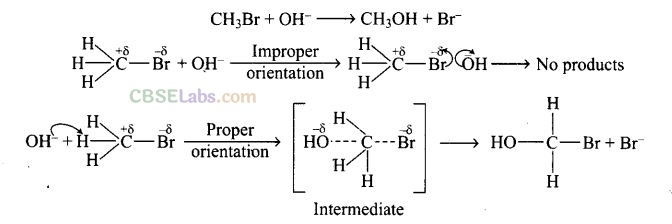The proper orientation of reactant molecules lead to bond formation whereas improper orientation makes them simply back and no products are formed. To account to effective collisions, another factor P (probability or steric factor) is introduced K = Ae-Ea/RT .

Question 63. What happens to most probable kinetic energy and the energy of activation with increase in temperature?
Solution: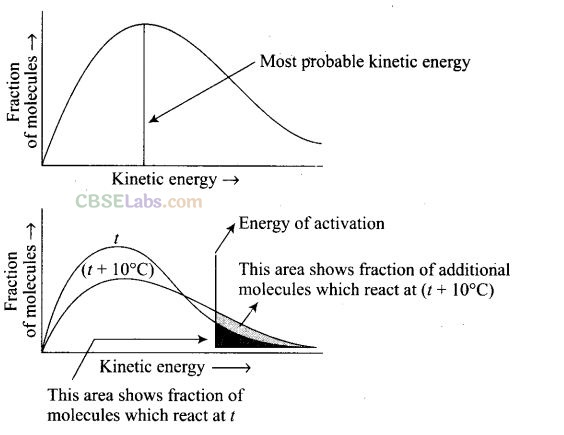When the temperature is raised’ the maxima of the curve moves to the higher energy value and the curve broadens out so that there is a greater proportion of the molecules with much higher energies. Most probable kinetic energy increases with increase in temperature. As the temperature increases, activation energy decreases which will result in the increase in the rate of reaction.

Question 64. Describe how does the enthalpy of reaction remain unchanged when a catalyst is used in the reaction?
Solution: A catalyst is a substance which increases the speed of a reaction without itself undergoing any chemical change.
According to “intermediate complex formation theory” reactants first combine with the catalyst to form an intermediate complex which is short-lived and decomposes to form the products and regenerating the catalyst.
The intermediate formed has much lower potential energy than the intermediate complex formed between the reactants in the absence of the catalyst.
Thus, the presence of catalyst lower the potential energy barrier and the reaction follows a new alternate pathway which require less activation energy. We know that, the lower the activation energy, the faster is the reaction because more reactant molecules can cross the energy barrier and change into products.
Enthalpy, AH is a state function. Enthalpy of reaction, i.e., difference in energy between reactants and product is constant, which is clear from potential energy diagram.
Potential energy diagram of catalysed reaction is given as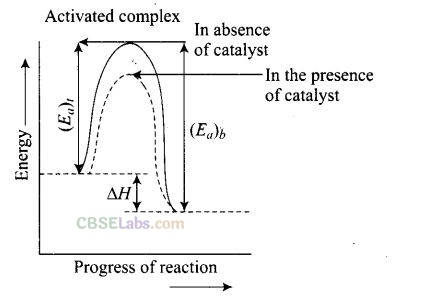Question 65. Explain the difference between instantaneous rate of a reaction and average rate of a reaction.
Solution: Average rate of a reaction is the change in concentration of reactants or products and the time taken for that change to occur.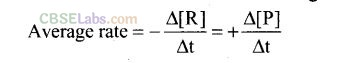It occurs for a long interval of time. It can be determined for multistep as well as elementary reactions.Question 66. With the help of an example explain what is meant by pseudo first order reaction?
Solution: A reaction in which one reactant is present in large amount and its concentration does not get altered during the course of the reaction, behaves as first order reaction. Such reaction is called pseudo first order reaction.
For example, (i) hydrolysis of ethyl acetateWe hope the NCERT Exemplar Class 12 Chemistry Chapter 4 Chemical Kinetics help you. If you have any query regarding NCERT Exemplar Class 12 Chemistry Chapter 4 Chemical Kinetics, drop a comment below and we will get back to you at the earliest.